Relative Density Of SoilA soil in its dense state has very little air voids present into it. If a soil in such dense state is applied with heavy structural loads it can take them with very little settlement, as there is very little space available for soil particles to settle into.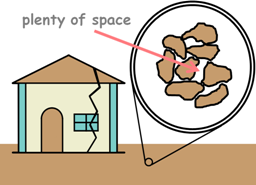But if the same soil is in loose state, there is plenty of space available for particles to move and settle down. It is undesirable for a structure to settle more than its permissible limits because it may generate problems of cracking and perhaps structural failure.

So prior to any construction we try to determine the denseness of the natural soil.

The degree of compaction of a soil is measured in terms of dry unit weight, which is the amount of soil solids that can be packed in a unit volume of the soil.

The unit weight of a soil provides an idea about its strength, compressibility, and permeability. Higher unit weights propose higher strengths and lower compressibilities and lower permeabilities.

But if two different soils have the same unit weights, they may produce different engineering properties. So there is a parameter which defines the degree of denseness of the coarse grained soil which is called Relative density.

Properties of soil that help in identification and classification of soil are called Index Properties. Among all the index properties, relative density is the most important property of the cohesionless soil.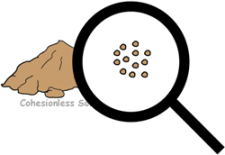Cohesionless soils are those which particles do not stick to each other. These soils are generally coarse grained soils, such as gravel and sand.

Relative Density, which is also called Density Index, is denoted as Dr and ID. It is the degree of denseness or looseness of natural deposits of coarse grained soils.

Mathematically it is defined as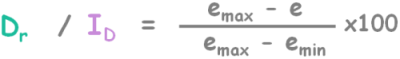Here e is the void ratio of soil at the field conditions. We have learned void ratio to define as volume of voids divided by volume of solids.

emax is the maximum void ratio of the same soil, which can be obtained if all the soil particles occupy the loosest condition. In this condition soil has maximum amount of volume of voids.

Similarly emin is the minimum void ratio of the same soil, when it is in its densest form.

In the loosest state relative density will be zero, because value of e will be equal to emax.

And in the densest condition it will be 100% as e will be emin.

On the basis of Relative density coarse grained soils can be divided into 5 categories.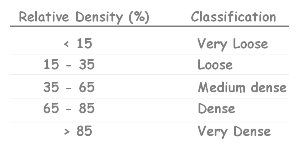So how do we determine the relative density values? Well, in this formula we see void ratios at different denseness of soil. But it is not easy to determine the void ratio of these samples because it is difficult to measure the volume of solids. But it is convenient to express void ratio in terms of dry unit weight.

We know this relationship.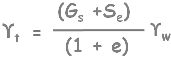For the dry mass of the soil saturation will be zero. So after rearranging a little we can find the void ratio in terms of dry unit weight.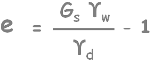Substitute this in the relative density equation and solve a little to represent Relative density in the form of dry unit weight.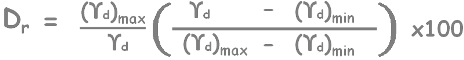Now we can determine the dry unit weight of differently densed soil masses experimentally as following.

We take the oven dried soil sample. If it contains bigger lumps then we pulverize it.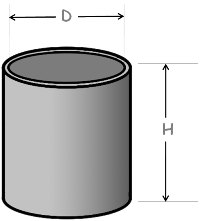Then we take a metal mould. Its height and diameter are noted down to calculate its volume. Mould is weighed and weight is noted as W1.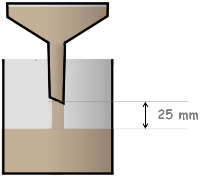Then we pour the dry pulverized soil into the mould using a pouring device. It should be held this way that height of the free fall of the soil is always 25 mm. This way it is considered that soil is in its loosest condition.

We over fill the mould and using some rod or plate soil surface is leveled up to the brim.

This loosely filled soil mould is weighed again and weight is noted as W2.

Now remove the soil content from the mould and attach the sleeve of the mould and fill the soil up to the sleeve's brim. Place this arrangement on a vibrator deck and place a surcharge load on the soil surface. Then vibrate it for 8 mins.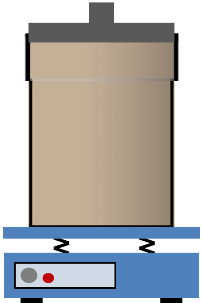If soil’s top surface falls below mould's brim we add more soil and vibrate it for another 8 mins.

This way soil can be obtained in compacted state which has low volume of voids.

Remove the sleeve and trim the soil surface. Then take the weight of this mould again and say it as W3.

Now by using all these measured weights and volume,

W1 = weight of mould

W2 = weight of mould and soil in loose state

W3 = weight of mould and soil in dense state

V = volume of mould

We can calculate maximum and minimum dry unit weight as weight of soil in different conditions and divided by volume of mould.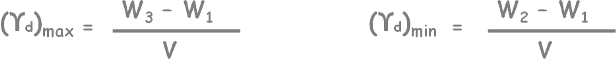Now in the equation of relative density, only unknown is the dry unit weight of soil in its natural conditions. We can calculate it by either Core Cutter Method or Sand Replacement Method.

Substituting all the values in the formula, relative density can be calculated and hence degree of denseness of coarse grained soil can be estimated.Relative density for coarse grained soils is equivalent to the relative consistency of clay soil.

Tags : relative density

Published on :2019-09-06